# Gravimetric analysis is more sensitive

The Gravimetry is a quantitative analysis method in which the measurement of substance quantities is based on the determination of the mass (weight). The ions or molecules are brought into a form of precipitation. The precipitated compound is filtered off, washed and dried. In some cases, the precipitation form is converted into a stoichiometric weighing form by annealing in specially designed ovens (muffle ovens), with which the quantitative determination of the ingredients can be carried out.

Sometimes the form of precipitation and weighing are identical. This is particularly the case when the precipitate has a clear stoichiometry and, for example, no changing amounts of water of crystallization are bound: the determination of sulfate ions as barium sulfate, the precipitation of nickel with diacetyldioxime, the determination of potassium with sodium tetraphenylborate. An example in which the precipitation form and the weighing form are not identical is the determination of iron as iron (III) oxide listed below.

### example

Determination of the iron content of an Fe (III) salt solution

The iron salt solution is mixed with ammonia water, the precipitated hydroxide (precipitated form) is filtered off and then converted into iron (III) oxide by annealing to constant weight. The mass of the oxide is determined by weighing it on the analytical balance.

Reaction equation: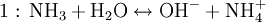(Basic reaction of ammonia)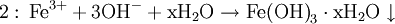(Precipitation form)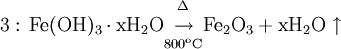(Weighing form)

The desired mass a of the element to be determined (in our example iron) is proportional to the balanced mass A of the weighing form (here Fe2O3). The proportionality factor λ indicates the proportion of the mass a in the balanced mass A.

From the balanced mass Fe2O3 Multiplication by a factor λ gives the mass a of the element to be determined.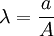or.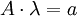.

On the one hand, λ contains the ratio of the molar masses of the element to be determined, M.s, to the molar mass of the weighing form, M.w. In addition, however, λ must also take into account "how often" a formula unit of the substance you are looking for is ultimately found per formula unit in the weighing form. In this example there are 2 iron atoms per formula unit of the weighing product. This is expressed by the stoichimetic coefficient k, here k = 2: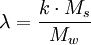where k is the stoichiometric coefficient, M.s the molecular mass of the substance sought and M.w is the molecular mass of the weighing form.

In our example we assume we have 1.25 g Fe2O3 balanced. How much iron did our Fe (III) salt solution originally contain?

Insertion of

A = m (Fe2O3) = 1.25 g
k = 2
M.s = M (Fe) = 55.845 g / mol
M.w = M (Fe2O3) = 159.69 g / mol

results in:

a = m (Fe) = 0.874 g.

This means that our Fe (III) salt solution contained 874 mg of iron.

Determination of the barium content of a sulphate salt solution

The solution is acidified with hydrochloric acid so that it is about 1M in HCl. A 0.1 M barium chloride solution is added dropwise with stirring until no more precipitate forms at the dropping point. The precipitate is tempered on a sand bath overnight. The precipitate is filtered off with the aid of a porcelain filter crucible, washed first with water, then with ethanol and finally with ether. The precipitate is dried for 2 hours in a drying cabinet, then calcined in a muffle furnace at 600 ° C. until the weight is constant. As a rule, this does not take longer than 2 - 3 hours.

Reaction equation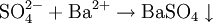(Precipitation and weighing form)

Category: Chemical analysis method